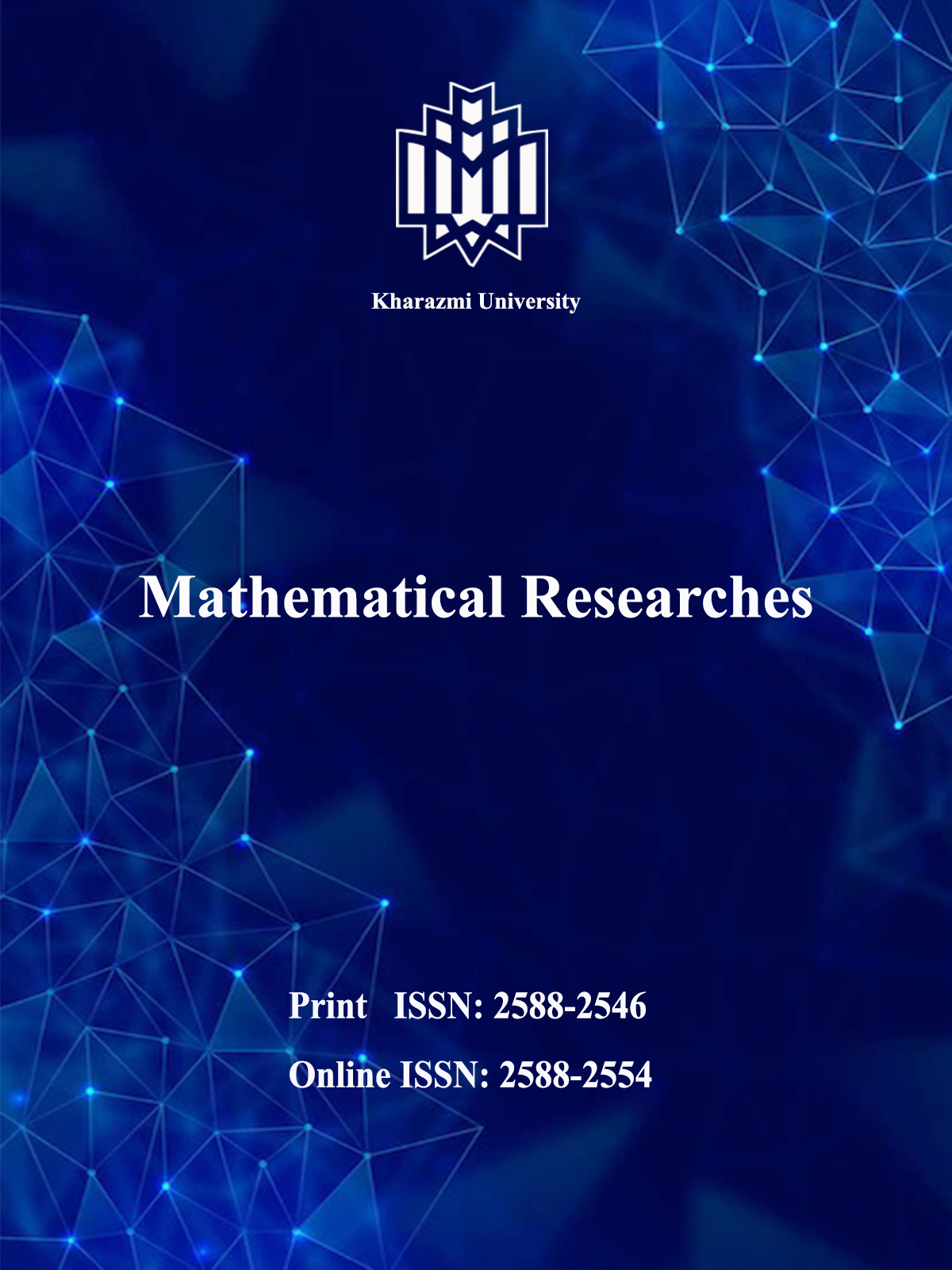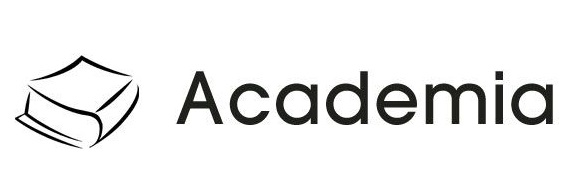[Home ] [Archive]   [ فارسی ]Search Submit ContactHomeJournal InformationArticles archiveFor AuthorsFor ReviewersRegistrationContact usSite FacilitiesEmblem Journal concessionaire  Kharazmi University Managing director Abdol-Javad Taherizadeh, Professor Editor-In-Chief Esmail Babolian, Professor  Manager Marjan Hamdollahi ISSN: 2588-2546 IISSN: 2588-2554Search in website
 Search the articlesSearch the site Advanced SearchEnter your email in the following box to receive the site news and information.Vol. 9, No. 1, 2023#### Mathematical Researches

ISSN Print:    2588-2546 | ISSN Online: 2588-2554
Publisher: Kharazmi UniversityMost visited published articles in last 6 monthsA numerical method for solving a class of distributed order time-fractional diffusion partial differential equations according to Caputo-Prabhakar fractional derivativeImproving clustering by detecting and removeing outliers based on Euclidean and exponential distance approachClassification of Krasner hyperrings(and new classes of hyperrings) of order less than four and their automorphismes groupsClassical and non-classical symmetries and analytical solutions of the system of fractional HGF differential equationsNumerical solution of the fractional optimal control problems by using Genocchi hybrid functions fractional operatorsFRACSION: A Novel Hybrid Algorithm for Forecasting the Industry Index Trend in Tehran Stock ExchangeOrbit space of cohomogeneity two actions on Riemannian manifolds of constant negative curvatureCategorical Relation between Dimension Groups, C*-algebras, and Cantor Minimal SystemsGrobner bases of determinantal idealsNumerical solution of random Ito-Voltaire integral equation with random polynomials using modified and improved cap base functionsFundamental groupoid and Whisker topologyDesign and Prototyping of the Driver Fatigue Detection System Based on Fuzzy Analysis of the Environmental Sensors DataUsing generalized confidence Intervals to compare two processes capability indicesOn fundamental hypergroups and their applications in fundamental particles physics and nuclear physicsAims| Post date: 2019/08/7 | Aims and Scope The journal of Mathematical Researches (MR) aims to publish high-quality original research articles that make a significant contribution to the research areas of both theoretical and applied mathematics. The journal does not have a length restriction and encourages the submission of longer articles in which more complex and detailed analysis and proofing of theorems are required.  It also publishes shorter research communications (Letters) covering nascent research in some of the hottest areas of mathematical research. The journal of Mathematical Researches (MR) publishes high-quality papers in all of the traditional areas of applied and theoretical areas of mathematics, and it actively seeks to publish seminal papers in the most emerging and interdisciplinary areas in all of the mathematical sciences. The journal of Mathematical Researches (MR) wishes to lead the way by promoting high-quality research of this type. The aim of the journal of Mathematical Researches (MR) is to provide fast publication of refereed, high-quality original research papers in all areas of applied mathematical sciences. This is a broad-based journal covering all branches of mathematics and interdisciplinary research. The journal of Mathematical Researches (MR) publishes research articles that treat scientific problems using methods that are of mathematical interest. Topics of interest include, but are not limited to: Actuarial science.  Algebra and number theory.  Applied mathematics.  Approximation theory and representation theory.  Computational mathematics.  Continuous modeling control theory.   Cryptography.  Differential equations (ODEs and PDEs).  Financial mathematics.  Game theory.   Graph theory.   Information theory.  Linear and nonlinear programming.   Mathematical analysis.  Mathematical biology.   Mathematical economics.   Mathematical methods of engineering.   Mathematical physics.   Mathematics.  Matrix theory.  Numerical analysis.   Operations research.  Optimization.   Probability.   Scientific computing.   Statistics.   Stochastic systems theory.   Theoretical computer science.Vol. 3 No.2 Autumn 2017&Winter 2018.User statistics :. Registered users: 1651 :. Online users: 0 :. Guest users: 7Visits statistics All visits: 2144882 Visits in 24 Hours: 610